In today’s lab we will use a number of different clustering and network building tools to explore interactions in a biological data set. These are “higher order” tools in the sense that they are suited to exploring complex data once all of the pre-processing has been done (normalization, handling of replicates, etc.) and can be used to analyze virtually any kind of underlying systems-level data (phylogenetic comparisons, functional genomics, proteomics, and others).

For illustration today, we’ll be using a smallish data set that nonetheless features a number very interesting interactions. Wilmes, et a. 2009 describes the construction of a high-density genetic interaction network (or E-MAP for epistatic mini-array profile).

In this study, we assembled a collection of 552 yeast strains, each carrying a loss-of-function mutation in a different gene. Target genes were picked for this collection based on known or implied roles in eukaryotic gene expression and mRNA processing (chromatin regulation, transcription, splicing, 5’ capping, 3’ poly-adenylation, export from the nucleus, mRNA degradation, and translation). Each of these strains was then crossed pair-wise with every other strain in the collection to make the full matrix of double mutant haploid progeny (~300k in total). Growth of each double mutant was then quantified by pinning the strains onto agar plates, taking pictures of the colonies, and using automated image analysis to measure colony size.

Tomorrow in class we’ll discuss the underlying data preprocessing and quality control procedures used in this study (Collins 2006), but for today we’ll just focus on the final data-set:

Parse and load this data-set and take a moment to familiarize yourself with its structure. You’ll see that the genotypes for the two parent strains are listed across the columns and down the rows, respectively. The genotypes marked “DELETION” represent clean deletions of the ORF at each locus; the “DAMP” strains were made to test essential genes by incorporating a cassette in the 3’UTR region that, on average, descreases the expression level of the transcript (“dampening” the levels of the encoded protein).

Step 2: Explore the raw data

It’s always a good idea to start a data analysis project with a quick look at the distribution of raw values in the data set you’re working with. In this lab, I’ll be showing example output plots, but leave it to you to work out an implementation strategy.

We can either use the built in density and plot functons, or the ggplot2 density geom to visualize the overall distribution of genetic interaction values across this entire data set (remember, the file is currently formatted as a pair-wise “matrix” of values).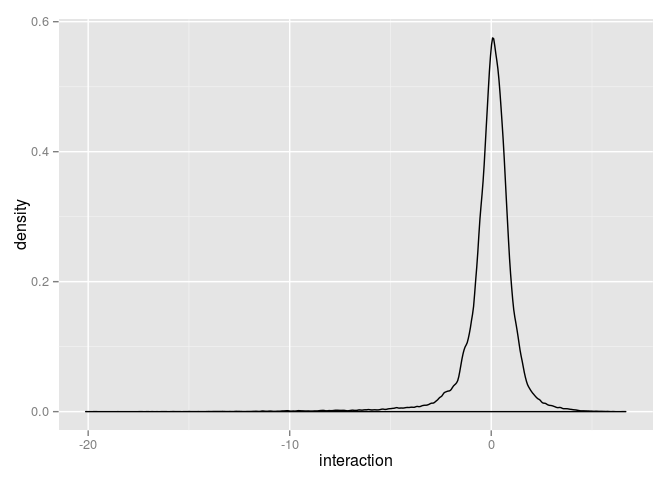We can clearly see that the data have been preprocessed resulting in a distribution of genetic interaction scores centered on 0; we can also see that there is a very long tail representing “synthetic” interactions (negative values). These data represent double mutants that are significantly “sicker” (or slower growing) than most; likely the result of two mutations that fall in the same multi-protein complex or functional module.

As we saw in last week’s lab, a hierarchical cluster is an easy way to get an initial peek at the structure of a large dataset. Here are a few tricks for tweaking the clusters you make using the builtin heatmap function:

• Use scale = "none" to suppress automatic data scaling across rows/columns. You should do this when working with this data set, since the values have already been appropriately normalized.

• Create a custom color series using the colorpanel function from the gplots and pass in your custom color series using the col argument. For example, one of my favorite color series is blue-black-yellow:

library(gplots)
heats <- colorpanel( n    = 500
, low  = "royalblue3"
, mid  = "black"
, high = "yellow"
)
• Use labCol = "" and labRow="" to suppress column and row labels, respectively, when your dataset is too large for these to be readable.

• Use na.rm = FALSE to suppress removal of missing values. Play with the effects inclusion or exclusion of missing values on the overal shape of your clusters.

• Use zlim = c(-5,2) (min value, max value) to change the point at which colors are saturated (in this example any valu <= -5 is the bluest-blue and any value >=2 is the yellowest-yellow).

• Use the distfun argument to play with different ways of computing node distances (as you’ll see, the default is to use dist with it’s default of euclidean).

• Use the hclustfun argument to play with different ways of assembling the cluster nodes (the “agglomeration” method).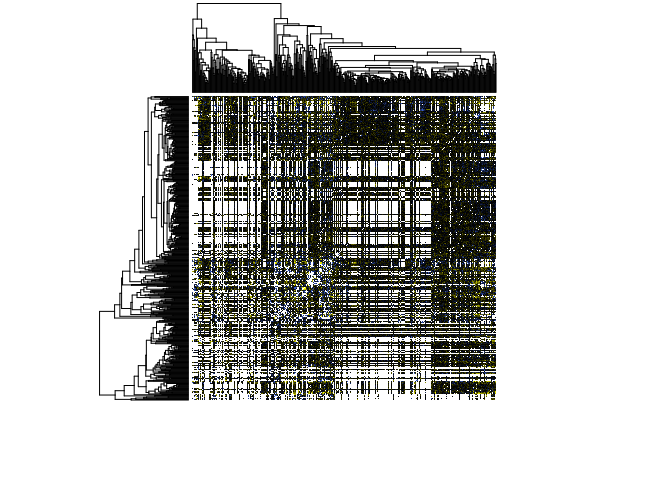We can see that the overall structure of this dataset is significantly different than the gene expression data from DeRisi et al. 1997. One of the most notable differences is the propensity of missing values. Values are missing if the double mutant strain failed to grow, or replicates failed to pass a quality control cutoff (see Collins et al., 2006).

Second, most distance and clustering methods will produce a set of dendograms revealing a much more granular overall topology of interactions than we saw last week (for example, it’s very hard to look at these trees and know where the right place to “trim” would be). Clearly, the data is being ordered in interesting ways when we cluster on the raw genetic interaction scores, but the patterns are a bit hard to clearly discern.

Step 3: Correlation matrix

As we saw in the yeast proteomics paper in class yeasterday, one approach to trying to find clear patterns in a complex data set is to calculate a correlation matrix from the raw data and then apply pattern recognition to the correlation matrix. In this case our raw data are the genetic interaction scores for each double mutant strain; the correlation matrix would describe how similar the score profiles for two different parental strains. For example, we can ask how well the genetic interaction profile for NPL3 (a 552 element vector) correlates with that of SKY1 (a second 552 element vector).

Fortunately, calcuating a correlation matrix is a common enough task that that aptly named cor function does it by default when passed a matrix or data.frame. Looking at the help page for cor you’ll see that we’re going to need to use the 'pairwise.complete.obs' option for the use argument, or we’ll end up with a matrix of all NA. For example:

interactionMatrix <- cor(emap, use = 'pairwise.complete.obs')

You’ll find it’s pretty hard to visualize the distribution of values in a large matrix like this; but we already know how to solve this problem: a heat map!

Since you’ve already seen how to do this using the built in image function, I’ll show you an attractive alternative, the tile geom from ggplot2. ggplot expects all data to be formated in a data.frame (and we have a matrix). The melt function for the incredibly useful reshape package does exactly what we want. For example:

library(ggplot2)
library(reshape)
##
## Attaching package: 'reshape'
##
## The following objects are masked from 'package:reshape2':
##
##     colsplit, melt, recast
interactionTable            <- melt(interactionMatrix)
colnames(interactionTable)  <- c( "parental.genotype.1"
, "parental.genotype.2"
, "value"
)

ggplot( interactionTable
, aes(x = parental.genotype.1, y = parental.genotype.2, fill = value)
) + geom_tile()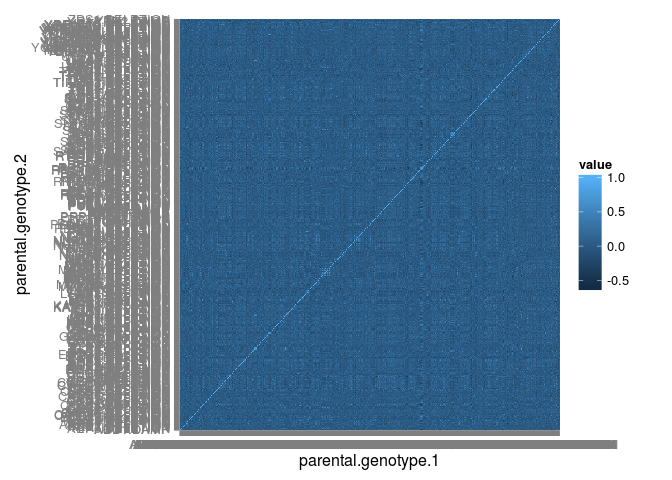As expected, when the rows and columns are unordered there aren’t many obvious groups (although, you’ll notice that the order of genes in the array wasn’t totally random!).

We can zoom in (the first set of 10 pair-wise correlations):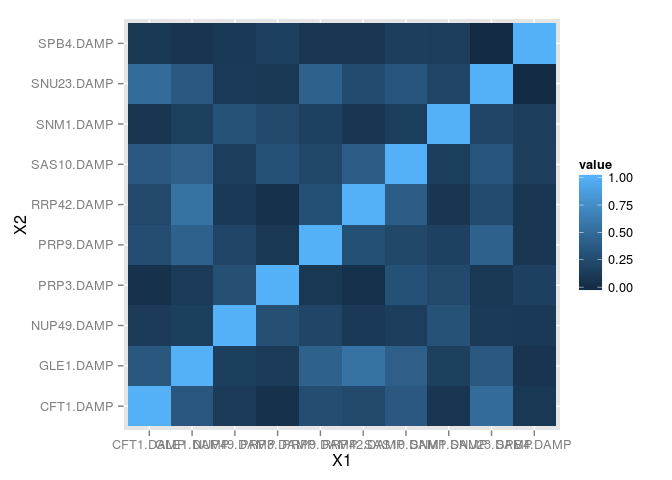We can spot check pair-wise correlations using scatter plots of the original raw data, for example: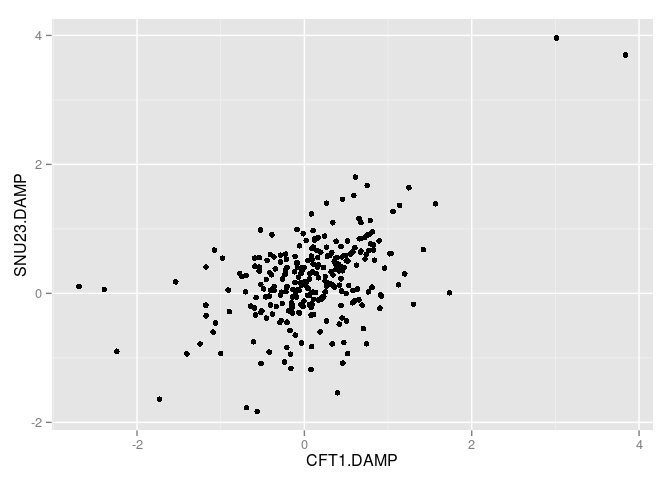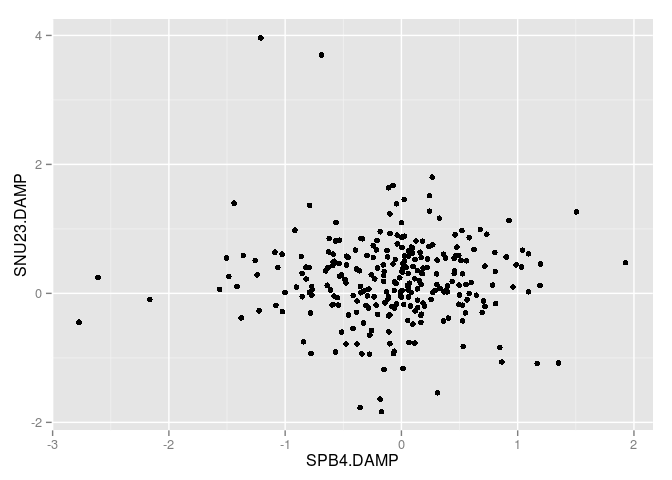Now experiment! Use the correlation matrix to perform either hierarchical clustering or kmeans cluserting. Explore the efffects of using different methods to calculate the correlation matrix (the default is pearson).

Challenges

If you’ve arrived here before the end of lab today, have a look at the following packages we’ll be using on Thursday:

• igraph for network building
• prcomp and princomp for principle component analysis